GET THE APP

Computational Study of Ailerons in Cross Flows Ground Effects and Biplanes Configurations# Journal of Aeronautics & Aerospace EngineeringOpen Access

Research Article - (2016) Volume 5, Issue 1

# Computational Study of Ailerons in Cross Flows Ground Effects and Biplanes Configurations

Alsarheed M* and Sedaghat A
Department of Mechanical Engineering, Australian College of Kuwait Mishref, Kuwait
*Corresponding Author: Alsarheed M, Department of Mechanical Engineering, Australian College of Kuwait Mishref, Kuwait, Tel: 965 1828225 Email:

## Abstract

An aileron, part of the trailing edge of a fixed-wing airplane, is used to control aircraft’s movement around its longitudinal axis (roll). Ailerons have significant impacts on airfoil surface pressure and its lift and drag coefficients. Both panel and finite volume methods were used on a NACA 2412 airfoil with a 20% aileron in a cross flow. The aerodynamic performance of ailerons alone, in a biplane configuration, and in the ground effects has been computationally investigated using both the panel and the finite volume methods. Several parameters were analyzed including the effects of the attack angle of the airfoil, aileron deflection angle, the ride height from the ground, and the characteristics of biplanes. Results of both computational methods are presented and discussed for the aforementioned configurations of NACA 2412 airfoil with aileron.

Keywords: Aileron; Biplane; Finite volume; Ground effect; NACA 2412; Panel method

#### Introduction

From flying a kite to operating a hypersonic vehicle, achieving and maintaining a high lift is always critical to a successful performance of a flying craft. Some of the advantages of an efficient lift system include improved maneuverability, higher wing payload capacity, longer range for given gross weight, and lower takeoff and landing speeds. Wing components such as trailing edge flaps play a major role in altering lift in conventional fixed-wing aircrafts. They control lift and extend speed range by upward or downward movements causing an increase or decrease in lift. Also, the flap deflection can reduce efficiency by causing or increasing drag, which sometimes is difficult to reduce due to flow with high Reynolds number. Part of the trailing edge assembly is a flap called ailerons, which is a French word for ‘little wing’ that is used to control the aircraft in its longitudinal axis (roll). This movement is called ‘rolling’ or ‘banking’ and it alters the flight path due to the titling of the lift vector. One of the main components that impacts lift is the airfoil. An airfoil is a cross sectional part designed to generate lift when it is subject to an air flow. Ailerons are not just limited to aircraft applications. They are also used in high speed levitation trains to control and maintain the hovering in track.

The airfoil configuration has been widely studied. There are several studies that optimize the airfoil configuration and assess the maximum airfoil lift capabilities [1,2]. Other literatures study the efficiency of the airfoil and the effects of different parameters on it . The camber and the Reynolds number effects have also been studied by many including Levy . Other work done by Birch  and Rinoie  include calculations of unsteady loading on the airfoil and the effect of trailing edge flaps on flow vortex shapes. Also, the performance of a wing in ground effect and the flow field characteristics has been studied in [7,8]. In addition, the lift distribution between biplane wings and other biplane characteristics have been looked at in . The objective of this paper is to investigate the performance and the ground effects on the ailerons of a biplane configuration. Both panel and finite volume methods were used on a NACA 2412 airfoil with a 20% aileron in a cross flow. Numerical measurements of surface pressure distributions were also obtained to determine the lift and drag coefficients for various configurations.

#### Airfoils Characteristics

For every action, there is an equal and opposite reaction. According to Newton's third law [10,11] an airfoil generates lift by diverting the motion of flow over its surface in a downward direction, resulting in an equal upward reaction. Typical airfoil geometry is shown in Figure 1.

The main parameters of an airfoil, which play a key role in its aerodynamic performance, are the angle of attack, the chord length, and the mean camber line. The chord is the straight line across the airfoil and it is used to measure the airfoil length. The mean camber is the line halved the airfoil thickness and it is used to measure airfoil curvature.

Common airfoil shapes have been characterized by the National Advisory Committee for Aeronautics (NACA) . Each airfoil shape is defined by this system by a series of digits that correspond to nondimensional airfoil properties. The number of digits series varies, but in this paper the focus is on four digit airfoils (NACA 2412).

A NACA 2412 airfoil has a maximum camber that is 2% of the chord length with a maximum thickness of 12% of the chord length, located 4/10 of the chord length away from the leading edge.

#### Computational Modeling

A NACA 2412 airfoil configuration with a 20% aileron was used for the panel and finite volume methods, and it is shown in Figure 2a. The chord length of the airfoil is designated by c, the velocity of the uniform flow is denoted by U, δf is the aileron deflection angle, and α represents the angle of attack. The flow is assumed to be two-dimensional steady and incompressible Laminar flow.

Figure 2a: NACA 2412 Airfoil with aileron, (b) Panels for NACA 2412 airfoil with 20% aileron divided into 70 panels.

Panel method

The airfoil was analyzed using a vortex panel method, where the airfoil was approximated by a finite number of panels. As shown in Figure 2b, the airfoil surface is divided into 70 panels. There are more panels near the leading and trailing edge to accommodate for the rapid changes in flow near these two ends. Each panel had local, uniform and distributed vortices. Since the velocities can be singular at the center of the vortex, and to avoid singularities, these vortices had infinitesimal strength γ0ds0, where γ0 is the vortex strength per unit length, and ds0 represents the length of a small segment of the airfoil.

The governing equation for the stream function due to all such infinitesimal vortices at a point in space may be given by: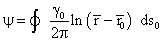(1)

where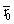is a point in the x-y plane with coordinates x0 and y0 and the distance between the position of the vortex, and the point where the velocity is evaluated with coordinates x and y is denoted by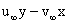. The x-y plane is originated at the leading edge of airfoil with x-axis along chord length and y perpendicular to it.

Equation (1) is integrated over all vortices on the airfoil surface and the stream function associated with the free stream is given bywhere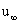and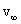are the x- and y- components of the free stream velocity. Taken these effects into account, the stream function at any point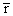in space is given by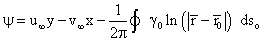(2)

Since the body itself is a streamline, the stream function value at all the points on the airfoil will be constant and the value is denoted by C. Also, for all points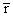on the airfoil surface, equation (2) becomes: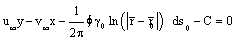(3)

The Kutta condition is an aerodynamics principle and it is applicable to the trailing edges of the airfoils. This condition states that both the pressure above and below the trailing edge of the airfoil must be equal. It also requires that the flow be smooth and must leave the trailing edge in the same direction at both upper and lower edges and it is expressed as follows: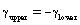(4)

For a finite number of N panels, there are equal N unknown vorticity strengths, denoted by γo, j, as shown in Figure 2(b). To approximate the line integral over the entire airfoil, it can be integrated as several line integrals over N panels, taking each value of γo as constant and so equation (3) becomes: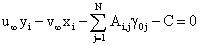(5)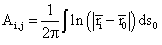Equation (5) can also be solved over the entire airfoil as several line integrals over the panels for the unknown vorticity strengths.

Finite volume method

The numerical simulations were conducted using FEMLAB software with a Reynolds number Re = 3 × 106. The Reynolds number Re is defined as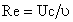(6)

Where U is the velocity of a uniform flow, c is the chord length of the airfoil, and υ denotes the kinematic viscosity

Where U is the velocity of a uniform flow, c is the chord length of the airfoil, and υ denotes the kinematic viscosity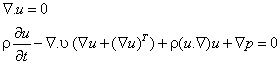(7)

Where ρ is the density, u represents the velocity vector, and p is the pressure.

#### Results and Discussions

Panel method

Aileron effects: For the zero aileron deflection case (δ = 0), the experimental results of NACA 2412 airfoil with data from Panel code and FEMLAB are compared in Figure 3. The results show a good agreement in all cases and validate the accuracy of the panel method.

Figure 3: Comparison of lift coefficient between Panel, FEMLAB and experimental data for a NACA 2412 airfoil.

Effects of a 20% aileron applied to the NACA 2412 airfoil on the value of lift force and moment coefficients are shown in Figure 4. Figure 4a shows the use of ailerons can change the lift over 50%. Also, by increasing the deflection angle, the drag coefficient increases rapidly. The moment coefficients for different aileron deflection angles are shown in Figure 4b and are based on 25% airfoil chord length. For a constant aileron deflection angle, the moment coefficient stays approximately constant for different attack angles.

Figure 4: (a) Force Coefficients for a NACA 2412 airfoil with aileron in cross flow (Panel Method), (b) Moment Coefficients for a NACA 2412 airfoil with aileron in cross flow (Panel Method).

Figure 5 shows variation of pressure coefficients for different deflection angles and an incidence angle of α = 3°. When the aileron is upward, the pressure coefficient has a considerable change approximately at the middle of the airfoil. For δf ≠ 0 around 0.8 airfoil length, there are two pressure peaks at the lower and upper surfaces of the airfoil. As it is shown in the figure, the deviation of these pressure peaks from the initial case (δ = 0) is larger when the aileron is downward.

Ailerons in ground effects: Fundamental geometric parameters such as ground clearance, wing camber and its thickness ratio can have impact on the performance of a wing in presence of a solid wall (ground). In Panel method, a wing in ground effect is equivalent to a two-wing case, where the second wing is the mirror image of the first, as shown in Figure 6a, and the wing-body combinations are solved simultaneously. The effect of ride height on the lift coefficient is presented in Figure 6b. The tests were performed at an angle of attack of 3°. At a close proximity of the wing to the ground, the effect of higher down force coefficients is clearly noticeable.

Figure 6: (a) Ground effect (Panel method) (b) Lift coefficients in ground effect for different ride heights (Panel method). (c) Lift coefficients in ground effect for different configurations (Panel method), (d) Pressure coefficients for a NACA 2412 airfoil without aileron (Panel method).

The physical effect of the ground is to constrain the airflow on the suction (lower) surface of the wing. This causes an acceleration of the flow compared with the case away from ground effects which results in a greater suction on the lower surface of wing and hence cause a higher lift force. As the ride height decreases, the ground effect causes the flow to be accelerated to a higher degree, generating a significantly higher lift force compared with free stream. At ride heights of less than 0.1c, there is a gradual and then significant deviation from the earlier trend of ever-increasing lift force with reduction in ride height. Eventually, the lift force falls off to reach a maximum CL of 2 at a ride height of 0.1c, as shown in Figure 6(b). The effect of attack angle on CL in ground effect is shown in Figure 6c. As it is shown, when the ride height is less than 0.15c, the lift coefficient decreases rapidly for α >10° as also observed in ref. . In Figure 6d, Cp distribution of a NACA 2412 without aileron is shown in ground effect. As it is expected, the pressure coefficient in this case only changes at the lower surface of the airfoil for different ride heights.

Figures 7a and 7b show the pressure coefficient (Cp) distribution when the aileron deflection of ± 20° is occurred in the airfoil. For δf = -20°, the pressure coefficient of the lower surface changes gradually due to the distance between airfoil and ground. It also decreases when the airfoil is closer to the ground. However, as it is shown in Figure 7b, when δf = +20° the pressure coefficient of the lower surface changes rapidly and reaches 8 at the connection of the aileron.

Figure 7: (a) Pressure coefficient distribution for a NACA 2412 airfoil with aileron in ground effect, α = 3° and δf = -20°, (Panel method) (b) Pressure coefficient distribution for a NACA 2412 airfoil with aileron in ground effect, α = 3° and δf = +20°, (Panel method).

Ailerons in biplanes: The effect of ailerons in biplane configurations on lift coefficient (CL) is examined using panel method. For a biplane configuration, the surfaces of two airfoils are divided into several N panels. The number of equations is equal to N+2 since there are N panels and (corresponding vortex strength) and two values of stream function C1 and C2 for the two airfoils. Also, there are two Kutta conditions, one for each airfoil. Then, the system of equations is set from influence of vortices on each panel and solved. Figure 8a shows the configuration of a biplane with aileron deflection, where the upper and lower airfoils are labeled as 1 and 2 respectively. The difference between aileron deflection in upper and lower airfoils and their effect on the lift coefficient are shown in Figure 8b and 8c. For a high aileron deflection and attack angle, the lift coefficient (CL) can reach as high as 4. According to the data shown in Figure 8(c), the choice of the airfoil for aileron deflection does not have significant effect on the lift coefficient for h/c >0.5. The pressure coefficient and the difference between aileron deflection of the upper and lower airfoils for α = 3°, δf = -20° and h/c = 0.5 is presented in Figure 8d. The results show that when the deflection is on the lower airfoil, the pressure coefficient of the upper airfoil has an unexpected distribution among the surfaces of the airfoil.

Figure 8: (a) Biplane configuration of NACA 2412 Airfoil with aileron (Panel method) (b) Lift coefficients in biplane with aileron deflection due to angle of attack (Panel method). (c) Lift coefficients in biplane with aileron deflection due to distance between the airfoils (Panel method) (d) Pressure coefficients in biplanes with aileron deflection in different configurations (Panel method).

Finite-volume method

Aileron effects: The results of NACA 2412 airfoil with data from FEMLAB, for the case where the aileron deflection is equal to zero, are shown in Figure 9. The experimental results and the results from the data generated from FEMLAB for NACA 2412 airfoil are in agreement.

Figure 9: (a) Lift coefficients-experimental and numerical data for a NACA 2412 airfoil (b) Drag coefficients-experimental and numerical data for a NACA 2412 airfoil.

Figure 10 shows the effects of a 20% aileron applied to the NACA 2412 airfoil on the value of lift and moment coefficients. From Figure 10a, it can be seen that the use of ailerons can change the lift over 50% but by increasing the deflection angle, the drag coefficient increase rapidly which is not desired. The moment coefficients for different aileron deflection angles are shown in Figure 10b and are based on a 25% chord length of the airfoil. As it is shown, for a constant aileron deflection angle, the moment coefficient stays approximately constant for different attack angles .

Figure 10: (a) Lift coefficients for a NACA 2412 airfoil with aileron in cross flow (FEMLAB) (b) Moment coefficients for a NACA 2412 airfoil with aileron in cross flow (FEMLAB).

Figure 11a and Figure 11b show the values of drag coefficient for different aileron deflection angles. The maximum drag can reach up to 0.24 for δf = - 60° when the lift coefficient is around 2.5, and it occurs when the aileron is downward.

Figure 11: (a) Drag coefficients for a NACA 2412 airfoil with aileron up in cross flow (FEMLAB) (b) Drag coefficients for a NACA 2412 airfoil with aileron down in cross flow (FEMLAB).

The difference of pressure coefficients for different deflection angles with α = 3° is shown in Figure 12. When the aileron is upward, the pressure coefficient has a considerable change approximately at the middle of the airfoil. Also, there are two pressure peaks at the lower and upper surfaces of the airfoil for δf ≠ 0. The deviation of these pressure peaks from the normal case (δf = 0) is larger when the aileron is downward.

The flow separation caused by different aileron deflections has also been analyzed. The outflow boundary condition is used at the downstream and the velocity at upstream boundary is selected to be the uniform velocity U. Figure 13a illustrates the airfoil-aileron configuration and the geometry surface grids. Figure 13b shows the effect of aileron deflections on the flow field at three aileron deflections, δf = -20, 0 and 20. When the aileron deflection is downward (δf < 0), the flow separation occurs earlier with more flow in the upward direction. Then, the flow reconnects again near the trailing edge when the attack angle is very small and forms a small separation region. When the aileron deflection is upward (δf > 0), the flow separation happens later with less flow occurs in the upward direction.

Figure 13: (a) Surface geometry and grids of a NACA2412 airfoil with 20% aileron, δf = -20° (b) Flow field velocity contours show separation patterns for different aileron deflections δf = 0, -20, 20, α = 20˚.

Ailerons in ground effect: Wing performance in presence of a solid wall (ground) is affected by some fundamental geometric parameters such as the ground clearance, the wing camber and its thickness ratio. Figure 14 shows flow field velocity contours of ailerons in ground effect.

Figure 14: Flow field velocity contours for NACA 2412 airfoil-aileron configuration in ground effect δf = ± 20°, α = 5°.

When the wing is in close proximity to the ground, the effect of higher lift force coefficients is noticeable. Figure 15a shows the effect of ride height on the lift coefficient at an incidence of 3°. The physical effect of the ground is to constrain the airflow over the suction surface of the wing. This causes an acceleration of the flow compared with the case out of ground effect and results in a greater suction on the suction surface and, hence, a higher lift force. As the ride height is reduced, the ground effect causes the flow to be accelerated to a higher degree, generating a significantly higher lift force compared with free stream. At ride heights of less than 0.1c, there is a gradual and then significant deviation from the earlier trend of ever-increasing lift force with reduction in ride height. Indeed, the lift force falls off, to reach a maximum CL of 2, at a ride height of 0.1c. The closer it is to the ground, the more lift force reduction is seen. The effect of attack angle on the lift coefficient (CL) in ground effect is shown in Figure 15b. When the ride height is less than 0.15c, the lift coefficient decreases rapidly for α >10°.

Figure 15: (a) Lift coefficients in ground effect for different ride heights (FEMLAB) (b) Lift coefficients in ground effect for different angles of attack (FEMLAB).

The effect of ride height on drag coefficient is shown in Figure 16a at an angle of attack fixed to 3°. As it is expected, when the airfoil is close to the ground, the drag coefficient is high and can reach up to 0.16 for a ride height of 0.1 and δf = - 40°. In Figure 16b, pressure coefficient (Cp) distribution of a NACA 2412 without aileron is shown in ground effect.

Figure 16: (a) Drag coefficients in ground effect for different ride heights (FEMLAB) (b) Pressure coefficients for a NACA 2412 airfoil without aileron (FEMLAB).

Figure 17a and 17b show the pressure coefficient (Cp) distribution when the aileron takes deflection angle of ± 20°. For δf = -20° case, the pressure of the lower surface changes gradually due to the distance between airfoil and ground and decreases when the airfoil is closer to the ground; but as it is shown in Figure 17b, when δf = +20° the pressure of the lower surface changes rapidly and reaches 8 at the aileron joint.

Figure 17: Pressure distribution for a NACA 2412 airfoil with aileron in ground effect, α = 3° (FEMLAB).

Ailerons in biplanes: In this section, the effect of ailerons in biplane configurations on the lift coefficient (CL) is examined. Figure 18a and 18b show the configuration and flow field for a biplane with aileron deflection. The difference between aileron deflection in upper and lower airfoils and their effect on the lift coefficient are shown in Figure 19a and 19b. For a high aileron deflection and attack angle, CL can reach as high as 4. Additionally, Figure 19b shows that the choice of the airfoil for aileron deflection has little or no significant effect on lift coefficient for h/c >0.5.

Figure 18: (a) Configuration of biplane (left) and (b) flow field velocity contours (right) for a biplane with aileron deflection.

Figure 19: Lift coefficients (using FEMLAB) in biplane with aileron deflection due to: (a) angle of attack; (b) distance between the airfoils.

#### Conclusions

The flow field over an airfoil with 20% aileron has been numerically examined using panel and finite volume methods. Many parameters play important roles in the efficiency of the airfoil. The effects of the attack angle of the airfoil, the flap length, flap deflection angle and Reynolds number are investigated in this paper. Also, viscous flows over the flapping airfoil with different flap combinations are computed. The results of both Panel and the Volume numerical methods are consistent are in agreement with the experimental results. The results also indicate that the existence of an aileron has significant impacts on the lift and moment of the airfoil. The use of ailerons in ground effect and biplane configurations relevant to levitated high speed train has been studied as well. The following conclusions are drawn:

• For the airfoil with aileron when the attack angle is increased, the lift is also increased by two folds.

• When the aileron is upward, the pressure coefficient has a considerable change approximately at the middle of the airfoil. There are two pressure peaks at the lower and upper surfaces of the airfoil for δf ≠ 0. The deviation of these pressure peaks from the normal case (δf = 0) is larger when the aileron is downward.

• When the airfoil-aileron configuration is close to the ground surface, force characteristics increase significantly and when the ride height is less than 0.15c, the lift coefficient decreases rapidly for α >10°.

• For the biplane case, the choice of the airfoil for aileron deflection has little or no important effect on lift coefficient for h/c >0.5. However, it is shown that when the deflection is on the lower airfoil, the pressure coefficient of the upper airfoil has an unexpected distribution among the surfaces of the airfoil.

#### References

Citation: Alsarheed M, Sedaghat A (2016) Computational Study of Ailerons in Cross Flows Ground Effects and Biplanes Configurations. J Aeronaut Aerospace Eng 5:161.

Copyright: © 2016 Alsarheed M, et al. This is an open-access article distributed under the terms of the Creative Commons Attribution License, which permits unrestricted use, distribution, and reproduction in any medium, provided the original author and source are credited.
Top